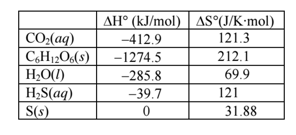# Problem: The process by which plants convert CO2 and H2O into C6H12O6 and O2 is called photosynthesis. This process is nonspontaneous, so energy must be provided to make the reaction occur, and that energy is provided by sunlight. “Chemosynthesis” refers to the biological conversion of simple carbon-containing molecules (e.g. CO2 or methane, CH4) into more complex energy-rich molecules using non-biological (inorganic) molecules as the energy source, rather than sunlight, as in photosynthesis. For example, giant tube worms found near deep-ocean sulfur vents contain bacteria that convert carbon dioxide to simple sugars using hydrogen sulfide as the energy source according to the chemical equation 12H2S + 6CO2 → C6H12O6 + 6H2O + 12S  A. Using the data provided below, calculate the following thermodynamic parameters for the above reaction.  1. ΔH°    2. ΔS°    3. ΔG°   B. Note the value you calculated for ΔG°. The normal expectation is that the reaction is spontaneous, but the calculations indicate that the reaction is nonspontaneous at 25°C.  At what temperature will the reaction become spontaneous?  When the reaction becomes spontaneous, will it be driven by enthalpy or entropy?

###### FREE Expert Solution
100% (463 ratings)
###### Problem Details

The process by which plants convert CO2 and H2O into C6H12O6 and O2 is called photosynthesis. This process is nonspontaneous, so energy must be provided to make the reaction occur, and that energy is provided by sunlight. “Chemosynthesis” refers to the biological conversion of simple carbon-containing molecules (e.g. CO2 or methane, CH4) into more complex energy-rich molecules using non-biological (inorganic) molecules as the energy source, rather than sunlight, as in photosynthesis. For example, giant tube worms found near deep-ocean sulfur vents contain bacteria that convert carbon dioxide to simple sugars using hydrogen sulfide as the energy source according to the chemical equation

12H2S + 6CO2 → C6H12O6 + 6H2O + 12S

A. Using the data provided below, calculate the following thermodynamic parameters for the above reaction.

1. ΔH°

2. ΔS°

3. ΔG°

B. Note the value you calculated for ΔG°. The normal expectation is that the reaction is spontaneous, but the calculations indicate that the reaction is nonspontaneous at 25°C.

At what temperature will the reaction become spontaneous?

When the reaction becomes spontaneous, will it be driven by enthalpy or entropy?What scientific concept do you need to know in order to solve this problem?

Our tutors have indicated that to solve this problem you will need to apply the Gibbs Free Energy concept. You can view video lessons to learn Gibbs Free Energy. Or if you need more Gibbs Free Energy practice, you can also practice Gibbs Free Energy practice problems.

What is the difficulty of this problem?

Our tutors rated the difficulty ofThe process by which plants convert CO2 and H2O into C6H12O6...as high difficulty.

How long does this problem take to solve?

Our expert Chemistry tutor, Ellen took 8 minutes and 28 seconds to solve this problem. You can follow their steps in the video explanation above.

What professor is this problem relevant for?

Based on our data, we think this problem is relevant for Professor Davis' class at UCF.